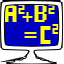⇦ | mathomatic [main]
Last updated on: 2022-01-24 08:16 [UTC]

mathomatic.desktop - 16.0.5-4 ⚙ amd64---
Type: desktop-application
ID: mathomatic.desktop
Package: mathomatic
Name:
C: Mathomatic
Summary:
C: Do symbolic mathematics and quick calculations
Description:
C: >-
<p>Mathomatic implements most of the rules of algebra for the mathematical operators +, -, *, /, % (modulus), and power
(including roots).</p>

<p>Mathomatic can symbolically:</p>

<p> * combine and solve equations,  * completely simplify and compare expressions and equations,  * do simple calculus
transformations and series,  * do standard, complex number, modular, and polynomial arithmetic,  * generate optimized
C, Java, and Python language code from    simplified equations,  * plot expressions with gnuplot in two or three dimensions,
* do quick calculations, colorize expressions, etc.</p>
en: >-
<p>Mathomatic implements most of the rules of algebra for the mathematical operators +, -, *, /, % (modulus), and power
(including roots).</p>

<p>Mathomatic can symbolically:</p>

<p> * combine and solve equations,  * completely simplify and compare expressions and equations,  * do simple calculus
transformations and series,  * do standard, complex number, modular, and polynomial arithmetic,  * generate optimized
C, Java, and Python language code from    simplified equations,  * plot expressions with gnuplot in two or three dimensions,
* do quick calculations, colorize expressions, etc.</p>
Categories:
- Education
- Science
- Math
Icon:
cached:
- name: mathomatic_mathomatic.png
width: 64
height: 64
stock: mathomatic
Launchable:
desktop-id:
- mathomatic.desktop